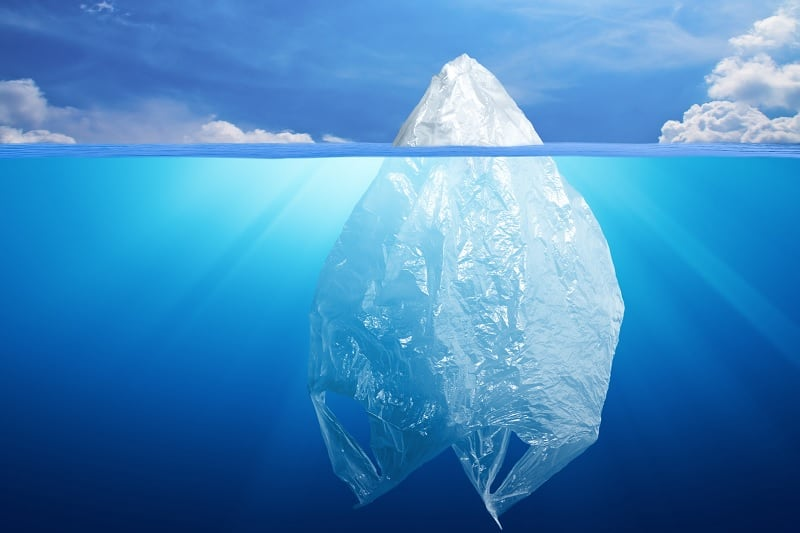# How Many Plastic Bags Are in the Ocean?It’s impossible to know exactly how many plastic bags are in the ocean. All we can do is piece different bits of data to get a very rough estimate.

According to Statista, total global plastic production was around 348 million metric tonnes in 2017 (source). Since we couldn’t find an updated figure for 2019, let’s assume this figure is the same.

• Fact #1: 348 million metric tonnes of plastic are produced a year

Next, we need to know how much of this plastic ends up in the oceans, and how much plastic is in the oceans overall.

According to Ocean Conservancy, about 8 million metric tonnes of plastic enter the oceans each year, and the total amount of plastic in the ocean is at least 150 million metric tonnes (source).

For simplicity, let’s assume that the total amount of plastic in the ocean is 150 million metric tonnes.

• Fact #2: 8 million metric tonnes of plastic enter the oceans each year
• Fact #3: The total amount of plastic in the oceans is 150 million metric tonnes

Based on these figures, roughly 2.3% of all plastic produced enters the ocean, and roughly 5.3% of the total plastic in the ocean is from the last year.

According to The World Counts, the world will consume 5 trillion plastic bags in 2019 (source).

• Fact #4: The world consumes 5 trillion plastic bags a year

If we assumed that 2.3% of these entered the ocean, that would be 115 billion plastic bags entering the ocean in 2019.

If 5.3% of the total plastic in the ocean was from the last year, this would mean that the total amount of plastic in the ocean would be roughly 18.9 times the amount that entered in the last year.

If we assume that plastic bags enter the ocean at the same rate as the average piece of plastic, this would mean that the total number of plastic bags in the ocean could be around 2.2 trillion.

• Conclusion: We estimate that there are around 2.2 trillion plastic bags in the ocean (in various states of decomposition)

It’s important to reiterate that this is a very rough estimate, and the true number could be much different.

Getting a very accurate figure for the number of plastic bags in the ocean is practically impossible.